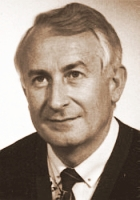Content Hotkeys

Variational Methods
CMS

# Workshop on Advances and Trends in Integral Equations

## The workshop is dedicated to the memory of Siegfried Prößdorf.Prof. Siegfried Prößdorf, 1939 - 1998

The honorific speech will be given by Bernd Silbermann.

# List of speakers

 H. Bart (Erasmus University Rotterdam) Vector-valued logarithmic residues and non-commutative Gelfand theory M. C. De Bonis (Universita della Basilicata, Potenza) Some Nyström methods for systems of Fredholm integral equations V. Didenko (Universiti Brunei Darussalam) On quadratic summable solutions of refinement equations J. Elschner (WIAS, Berlin) On uniqueness in inverse elastic scattering L. Fermo (Universita della Basilicata, Potenza) A discussion on the regularising parameter of some integral equations F. Filbir (Helmholtz-Zentrum München) Quadrature formulas for functions defined on Riemannian manifolds C. Frammartino (Universita della Basilicata, Potenza) A Nyström method for high order BVP in [-1,1] I. Gohberg (Tel Aviv University) The state space method for singular integral equations K. Gürlebeck (Bauhaus-Universität, Weimar) On the approximation of the solutions of some elliptic boundary value problems by monogenic polynomial Appell sets M. A. Kaashoek (Vrije Universiteit, Amsterdam) Canonical systems on a finite interval: accelerants and continuous potentials B. N. Khoromskij (MPI für Mathematik, Leipzig) Tensor-structured methods to approximate elliptic inverse in R^d G. Mastroianni (Universita della Basilicata, Potenza) Revisiting a quadrature method I. Notarangelo (Universita della Basilicata, Potenza) Fredholm integral equations on [-1,1] with exponential weights S. V. Pereverzev (Johann Radon Institute, Linz) Multi-parameter regularization and its numerical realization J. Prestin (Universität zu Lübeck) On exponentially localized kernels based on Jacobi polynomials A. Rathsfeld (WIAS, Berlin) Numerical solution of an inverse scattering problem by an elastic obstacle G. Schmidt (WIAS, Berlin) Boundary integral methods for periodic scattering problems R. Schneider (Technische Universiät Berlin) Optimization with tensor products of fixed rank W. Sickel (Friedrich-Schiller-Universität, Jena) Nonlinear approximation and function spaces of dominating mixed smoothness F. O. Speck (Instituto Superior Tecnico, Lisboa) On some connections between pure Hankel operators, extensions of Helmholtz solutions into conical Riemann surfaces and the factorization of a special matrix W. Sprößig (TU Bergakademie, Freiberg) Initial-boundary value problems and quaternionic analysis M. Tasche (Universität Rostock) An inverse problem of digital signal processing G. Vainikko (University of Tartu) Cordial Volterra operators and equations E. Wegert (TU Bergakademie, Freiberg) How to solve singular integral equations using frog spawn W. L. Wendland (Universität Stuttgart) Boundary integral equations for two-dimensional low Reynolds number flow past a porous body M. Yamamoto (University of Tokyo) Initial value/boundary value problems for fractional diffusion-wave equations and inverse problems

# Special Sessions

There will be three special sessions oganized by

S. Kunis (Approximative Fast Algorithms)

 Speaker: F. Martin (TU Bergakademie, Freiberg) Adaptive wavelet methods for computing the Hilbert transform Speaker: M. Espig (Max-Planck-Institut für Mathematik, Leipzig) The efficient treatment of high dimensional problems by use of elementary tensor sums Speaker: R. Hielscher (Helmholtz-Zentrum München) An algorithm for the fast evaluation of the spherical mean value operator Speaker: L. Kämmerer (Technische Universität Chemnitz) High dimensional sparse fast Fourier transform Speaker: J. Keiner (Universität zu Lübeck) The fast multipole method and the connection problem for classical orthogonal polynomials Speaker: V. Turunen (Helsinki University of Technology) Gaussian chirplets in time-frequency analysis of sounds
M. Lindner (Spectral Approximation of Linear Operators)
 Speaker: L. Boulton (Heriot-Watt University, Edinburgh) Weyl-type theorems for limit sets of the finite section spectrum Speaker: S. Chandler-Wilde (University of Reading) Spectral approximations and spectral inclusion sets for non-self-adjoint tridiagonal and band-dominated operators Speaker: R. Chonchaiya (University of Reading) Approximating the spectrum and pseudospectrum of a non-self-adjoint random tridiagonal operator A. Karlovich (Universidade Nova de Lisboa and CEAF, IST) Finite section method for a Banach algebra of convolution type operators on L^p(R) with symbols generated by PC and SO Speaker: M. Plum (Universität Karlsruhe) A computer-assisted proof for photonic band gaps Speaker: S. Serra-Capizzano (University of Insubria, Como) From Toeplitz matrix-sequences to generalized locally Toeplitz sequences
K. Rost (Analytical and Numerical Treatment of Structured Problems)
 Speaker: D. Bini (Universita di Pisa) Computing matrix geometric means Speaker: F. Bünger (Hamburg University of Technology) Minimizing and maximizing the Euclidean norm of the product of two polynomials Speaker: D. Lenz (Friedrich-Schiller-Universität Jena) Dirichlet forms and stochastic completeness of graphs and subgraphs Speaker: M. Seidel (Technische Universität Chemnitz) A Banach algebra framework for the finite sections of band-dominated operators Speaker: D. Wenzel (Technische Universität Chemnitz) Impressions of norm interpolation - An illustration for commutator bounds

# Registration

The registration is completed.

# Abstracts

For the abstract one can us this latex-file.

# Location

The workshop will take place at Hotel Wasserschloss nearby Chemnitz. The conference site Hotel Wasserschloss can be reached from the main station Chemnitz by City-Bahn using the station Neukirchen-Klaffenbach.

Navigate within this image or click here for a larger map of the conference site.

# Time

Monday, 5th of Oktober 2009 till Friday, 9th of Oktober 2009

As arrival date we prefer Sunday and as departure time Friday afternoon. The workshop will be terminated about 12:00 on Friday.